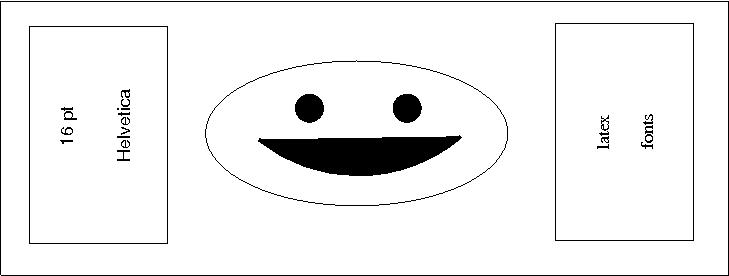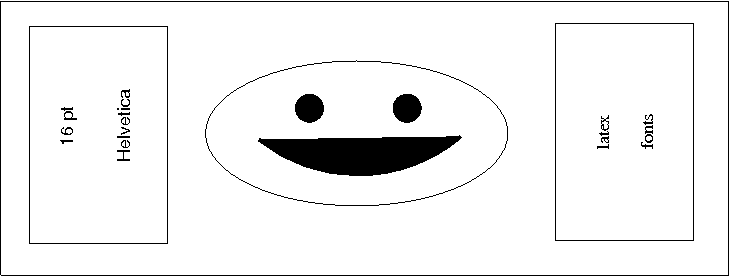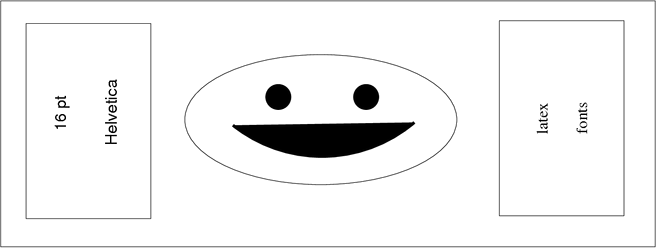## 7.3 Graphicx package

The following are the tested methods for including images of various formats which will enable the images to be available in the transformed versions. When using these methods we should be able to directly insert jpg (figure 3), png (figure 4) and pdf (figure 5) formats. The accepted method for working with eps format and psfrag in this context is also given below for reference.

For included graphics an alt tag should be defined. This should include a full description of the image for a screenreader user. For images included via the graphicx package a command \nextalt is provided in a local configuration file. This should be used directly before inclusion of the image.

All includegraphics commands must include the file extension and a value for the required angle and the width. No other rotation method should be used. All figure widths need to be in terms of a multiple of the current textwidth to ensure that large print formats can produce a realistic estimate of the necessary size of the image in the transformation. You must set the width before you alter the angle because angle alters the textwidth value.

\begin{figure}[!hptb]
\noindent
\nextalt{This is an alt text for the following image $4$!}
\includegraphics[width=\textwidth, angle=0]{smile1.jpg}
\caption{A jpg}\label{jpg}
\end{figure}

\begin{figure}[!hptb]
\nextalt{This is an alt text for the following png image $4$!}
\includegraphics[width=\textwidth, angle=0]{smile2.png}
\caption{A png}\label{png}
\end{figure}

\begin{figure}[!hptb]
\nextalt{This is an alt text for the following pdf image $4$!}
\includegraphics[width=\textwidth, angle=0]{smile3.pdf}
\caption{A pdf}\label{pdf}
\end{figure}Figure 3: A jpgFigure 4: A pngFigure 5: A pdf

### EPS and psfrag

You may not include EPS format directly. If you have EPS format graphics and cannot convert these because you need to use psfrag for the text then the following is the quoted, recommended strategy from “Using Imported Graphics in LaTeX  and pdfLateX” [4, p.49].

1. For each graphic that uses psfrag, create a separate LaTeX  file containing the psfrag commands and the \includegraphics command. You must use \pagestyle{empty} to prevent page numbers from being placed on the page.

Assume these psfrag LaTeX  files are named

GraphicFrag00.tex,
GraphicFrag01.tex,
...

2. At the operating system command line, perform the following steps:

latex GraphicFrag00.tex
dvips -E GraphicFrag00
epstool --copy --bbox GraphicFrag00.ps GraphicFrag00.eps
epstopdf GraphicFrag00.eps


The first command creates GraphicFrag00.dvi. The second command creates GraphicFrag00.ps. The third command calculates the BoundingBox for GraphicFrag00.ps and inserts the BoundingBox and contents of GraphicFrag00.ps into GraphicFrag00.eps. The last command converts GraphicFrag00.eps into pdf format.

3. Repeat step 2 for GraphicFrag01.tex, ...

4. Use \includegraphics to include the resulting pdf files GraphicFrag00.pdf, GraphicFrag01.pdf, ... into the original LaTeX  file.

5. Process the LaTeX  file with pdflatex.

In step 4 you should use the method given above for including PDFs. Please note that if you do this you will need to create large print versions of each PDF with the appropriate fontsize set using extsizes.